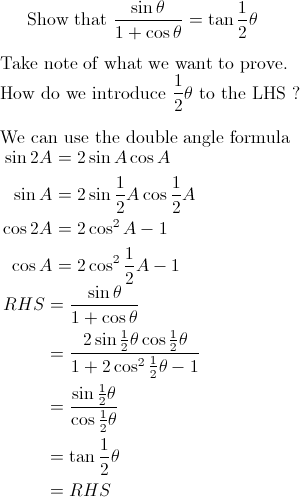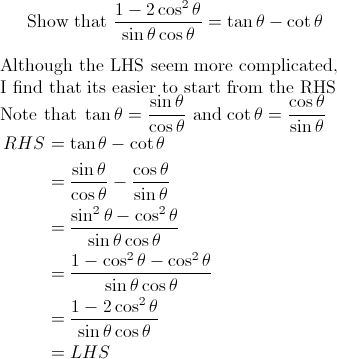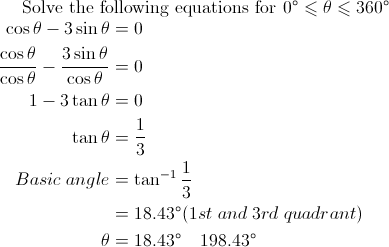## Posts

Showing posts from February, 2010

### Trigo Master Post

Basic Trigo Trigo Equations Part I Trigo Equations Part II Trigo Equations Part III Trigo Equations Part IV R formula Part I R formula Part II Proving Trigo identities Part I Proving Trigo identities Part II Proving Trigo identities Part III Proving Trigo identities Part IV

### Proving trigo identities Part IV### Proving trigo identities Part IIIThis question involves the use of rationalization to prove trigo identites Rationalization is a commonly used technique in surds. Click here to check out how rationalization is used in surds. Question 1 Whats Next? Click here for Proving trigo identities Part IV Click here for Contents Page

### Proving trigo identities Part IIQuestion 1 My friend came up with another way of proving the above identity Question 2 What's Next? Click here for Proving trigo identities Part III Click here for Contents Page

### Proving trigo identities Part IStrategy Choose the more complicated side to start Keep in mind what you are trying to prove Question 1 Show that: Solution:  Question 2 Show that The more complicated side is LHS What's Next? Click here for Proving trigo identities Part II Click here for Contents Page

### Trigo Equations Part IVEquations that contain only sin x and cos x Strategy: Divide by cos x  to obtain tan x This type of equation has to be solved using the R formula . What's Next ? Click here to learn how to trigo equations using the R formula Click here to learn how to prove trigo identities Click here for Contents Page

### Trigo Equations Part IIIFactorization to obtain basic type Question 1 Use of idenity followed by factorization Question 2 Question 3 What's Next? Click here for Trigo Equations Part IV Click here for Contents Page

### R formula Part IIABCD is a quadrilateral where AB = 2 cm and BC = 4cm. Angles BAD and BCD each equals to θ a) Express the length of AD in terms of θ b) Determine the maximum length of AD and the corresponding value of θ c) Find the value of θ for which AD= 3cm Worked solutions a) b) c) Given AD = 3 cm What's Next? Click here for Contents Page